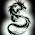# Multiplication Shortcut Techniques - Hareesh Kumar

Friends, here is a simple shortcut technique for multiplications shared by our Friend Hareesh Kumar. This technique can be used for smaller numbers i.e., for 2-digit numbers. Hope it helps. All the Best.

Note : This can also be applied for 3-digit or 4-digit numbers, but it makes a complex calculation.

Examples:
1)      12*18 =?
Sol: 12*18 = {1*(12+8)} | (2*8) = 20 | 16 = (20+1)|6 = 216     {Here ‘1’ becomes the carry}
Sol: 15*19 = {1*(15+9)} | 9*5 = 285
3)      24*28 =?
Sol: 24*28 = {2*(24+8)} | 4*8 = 64|32 = 672
4)52*57 =?
Sol: 52*57 = {5*(52+7)} | 2*7 = 295|14 = 2964
4)      12*24 =?
Sol: 12*24 = {[1*(24+2)] | 4*2} + (2*101) = 268 + 20 = 288
{1 – Difference between the 10’s digits of the two numbers}
5)      27*45 =?
Sol: 27*45 = {2*(45+7) | (5*7)} + (7*20) = (104|35) + (140) = 1075 + 140 = 1215
6)      33*67 =?
Sol: 33*67 = {3*(67+3) | (7*3)} + (3*30) = (210|21) + 90 = 2121+90 = 2211
7)      55*97 =?
Sol: 55*97 = {5*(97+5) | (7*5)} + (5*40) = (510|35) + 200 = 5135+200 = 5335

8)      121*132 =?
Sol: 121*132 = {1*(132+21)} | (32*21) = 153|672 = 15972
9)      154*226 =?
Sol: 154*226 = {1*(226+54) | (26*54)} + (54*100) = (280|1404) + 5400 = 34804
10)  443*503 =?
Sol: 443*503 = {4*(503+43) | (43*03)} + (43*100) = (2184|129) + 4300 = 218529+4300 = 222829

1.I think is take too much time to solve multiplication. This method is only suitable for multiplication of two digit numbers.

1.this method that works fast if u are asked to solve 90*12 consider 90 *10 =900 ,so step 2 ,12 is 2 more than 12 so 90*2=180 ,add 900 +180=1080...cheerz.....

2.this method that works fast if u are asked to solve 90*12 consider 90 *10 =900 ,so step 2 ,12 is 2 more than 12 so 90*2=180 ,add 900 +180=1080...cheerz.....

3.ya time taking process.......